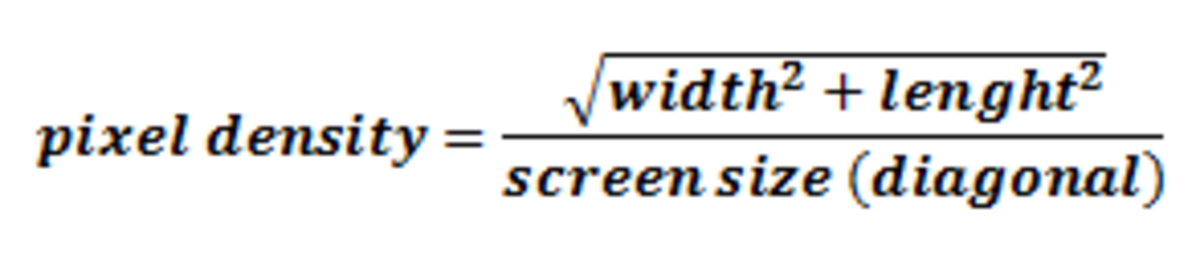# How to Calculate a Display's Pixel Density (ppi/Pixels Per Inch)

I'm a passionate informal educator, and I have a few years of experience as a high school teacher.

## What Is Pixel Density?

Pixel density is a metric telling us how many pixels there are in a fixed area of a display. It's a very important metric because it lets us know how closely packed the pixels on a display are. This is something that determines the quality, clarity, and readability of the image displayed. It is usually measured in a unit called pixels per inch (ppi).

Very often, when we are confronted with evaluating the quality of a display and/or comparing two or more displays together, we don't have all the information available. Something that is often missing is the pixel density, which is actually a very important metric and can provide us with valuable information about the picture quality we could expect. But we almost always know the size (diagonal) of the screen given in inches and the resolution. Having this information, we could easily calculate the pixel density ourselves.

## Calculation

If we have two displays with the same resolution, the smaller one will have the higher pixel density. And if we have displays with the same size, the one with a higher resolution will have the higher pixel density. So it's clear that the two parameters that we need in order to calculate the pixel density are display size and resolution.

## Pixel Density FormulaFigure 1. Calculating the pixel density knowing the resolution and size (diagonal) or a display

## Using the Right Units

You need to make sure you are using the right units in order to get the right result in the expected unit. If there are units that don't fit, they need to be converted.

• Resolution - number of pixels (usually not specially denoted)
• Display Size (diagonal) - inches
• Pixel Density - pixels per inch

First, you need to calculate the diagonal resolution and then divide it by the size (diagonal in inches) of the display. The diagonal resolution is the square root of the sum of the squares of the two multiples of the resolution (width and length). In order to make it sound and look easier to understand we can put this all together into a single formula that you can see in Figure 1.

If you don't have information about the diagonal of the screen, you can always calculate it using the Pythagorean theorem using its length and width. The diagonal is the square root of the sum of the squares of the length and the width. Diagonal = square root of (width2 + length2).

## Example 1 (Textual)

Let's say we have a smartphone with a 4.5-inch display and a resolution of 768x1280. It means that we have all the parameters we need to do the calculation: width=768; length=1280 and display size=4.5 inch.

First, we are going to determine the diagonal resolution by calculating the square root of 7682 + 12802. 7682 + 12802 = 589824 + 1638400 = 2228224 and the square root of that is about 1492.7. This is the diagonal resolution, which we now divide by the diagonal 4.5 inches and get the answer of roughly 332ppi, which is a really outstanding result for a smartphone.

## Example 2 (Mathematical)

Let's say we have a computer display that is 21.5 inches with a 1920×1080 resolution.

• Display size = 21.5 inches
• Width = 1920
• Length = 1080

### Diagonal Resolution

• Square root of (19202 + 10802)
• Square root of (3686400 + 1166400)
• Square root of 4852800
• 2203 (close approximation)

### Pixel Density

• Diagonal resolution/display size
• 2203 / 21.5
• 103ppi (close approximation)
Scroll to Continue

RAVI ASHAR on May 04, 2019:

How can we get device diagonal size in pixel

onesmo jason on August 27, 2018:

Sheraz Khalid on May 03, 2018:

Very nice and easy pice of information

Reshu Vaish on May 03, 2018:

How we do in case we don't know the display size ?

Sumit Singh on July 16, 2015:

Thanks a lot for taking the time out for explaining this. The article is extremely well written and lucidly explained. Great job.

jon on June 30, 2015:

Why do the vertical and horizontal resolutions have to be squared and then the square root of that taken?

Distant Mind (author) on May 26, 2014:

Thanks for pointing a possible mistake out. Where is the mistake though? Why do you think it's incorrect?

shq on May 22, 2014:

PIXEL DENSITY = 2203 / 21.5

this is incorrect.

Distant Mind (author) on October 08, 2012:

Well, I guess being shocked is a good state to open up one's mind for learning, isn't it? :)

Thank you for the nice comment teaches and I'm glad there was something new learned today.

Dianna Mendez on October 08, 2012:

I hear my tech students talking about this on occasion. Now, I can talk to then with understanding.. won't they be shocked!